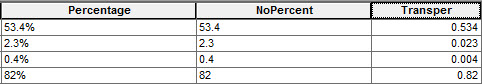English

# How To: Convert percentage fields to decimal fields

## Summary

When working with numerical data, the attribute table may contain fields with values in percentages, and these values are represented in numerical form with the % symbol attached. These fields are commonly of the Text data type, as the % symbol is special and only supported in Text data type fields. Attempts to convert the values of these fields to decimal involve transferring and converting the values to the Double data type field.

## Procedure

Below are the steps to convert a field with values in percentage to decimal. The workflow requires using the Field Calculator and involves adding two new fields to the attribute table.

1. In ArcMap, right-click the selected layer containing the values with percentage and click Open Attribute Table.
2. Start an edit session via the Editor toolbar.3. Create a new Double data type field (in this example, named NoPercent). This field stores the values without the percentage symbol. For more information, refer to ArcGIS Help: Adding fields.
4. Right-click the NoPercent field and select Field Calculator.
5. Perform the steps listed in How To: Remove and replace characters from a field in an attribute to replace the percentage with a blank space using the following sample code:
`![FieldName]!.replace("%"," ")`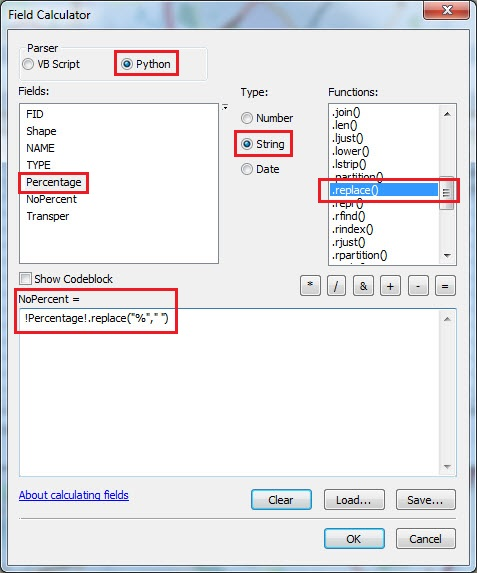The following image shows a sample output: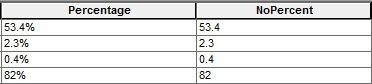6. Create a second field with the Double data type with a Precision of 15 (in this example, named Transper). This field is used to store the final conversion values. The following image shows a sample output: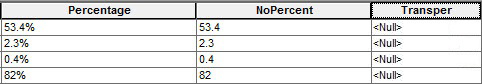7. Right-click the Transper field created in step 6 and select Field Calculator. Populate the field with the values from the NoPercent field.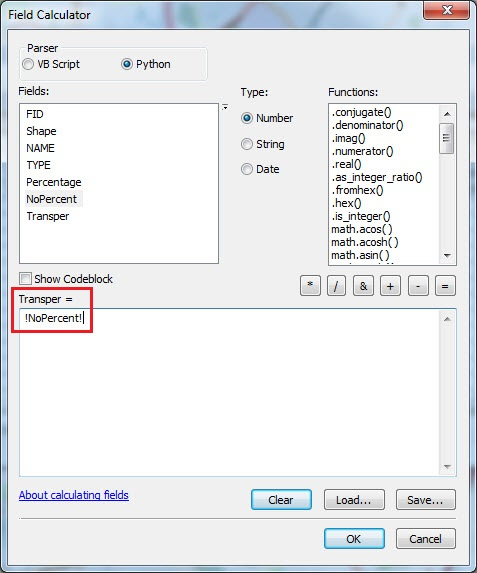The following image shows a sample output: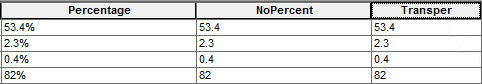8. Right-click the Transper field again and select Field Calculator.
9. Select Tranpser and multiply it by 0.01 to convert the values to decimal. For example:
`![Field Name]!*0.01`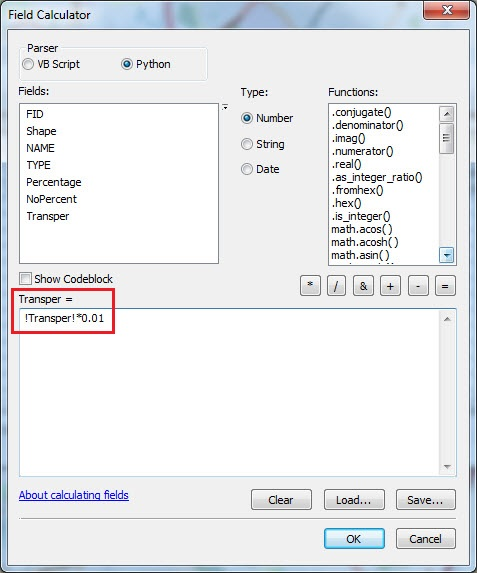The following image shows a sample output after a successful conversion: